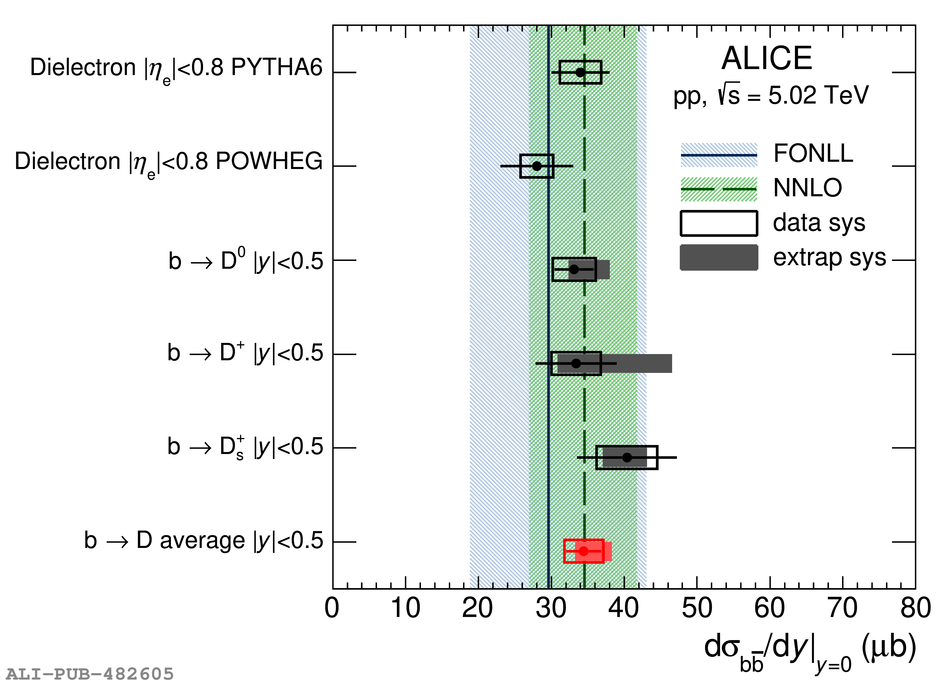# Figure 12

 < p>Estimates of $\de\sigma_\bbbar/\de y$ at midrapidity from dielectron~ and non-prompt $\Dzero$, $\Dplus$, and $\Ds$ meson measured in pp collisions at $\s=5.02~\TeV$ compared to FONLL~ and NNLO~ predictions. The average $\de\sigma_\bbbar/\de y$ of the estimates from the single D-meson species is also reported.< /p>